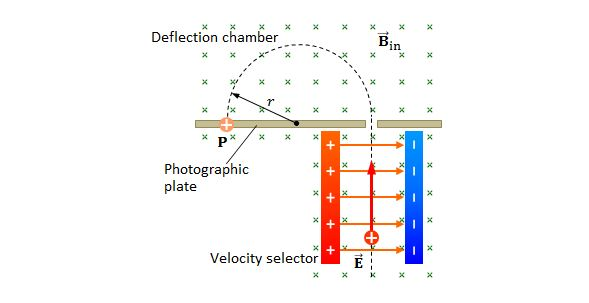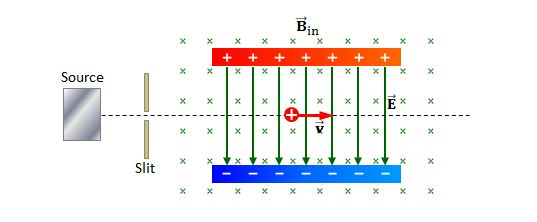Electricity and Magnetism

# Lorentz force law (mixed fields)

Consider a charged particle moving in a magnetic field with a constant velocity of $20\hat{i} \text{ km/s},$ in a direction perpendicular to the magnetic field. The mass and charge of the particle is $13\text{ g}$ and $75\,\mu\text{C},$ respectively. If the free-fall acceleration in the magnetic field region is $-9.8\hat{j} \text{ m/s}^2,$ what is the appropriate magnetic field?

A uniform magnetic field $\vec{B}$ of magnitude $1.5\text{ mT}$ is directed vertically upward. If a proton with kinetic energy $5.5\text{ MeV}$ enters the chamber horizontally, what is the approximate magnetic deflecting force acting on the proton as it enters the magnetic field?

The proton mass is $1.67 \times 10^{-27} \text{ kg}$ and $eV=1.60 \times 10^{-19} \text{ J}.$ (Neglect Earth's magnetic field.)The above is a schematic diagram of a mass spectrometer. The magnitude of the electric field between the plates of the velocity selector is $950 \text{ V/m},$ and the magnitude of the magnetic field (directed into the screen) in both the velocity selector and the deflection chamber is $0.910 \text{ T}.$ If a singly charged ion with mass $m=2.20 \times 10^{-25} \text{ kg}$ passes the velocity selector region with no deflection, what is the approximate radius of the path in deflection chamber?

The value of the elementary charge is $e=1.60 \times 10^{-19} \text{ C}.$In the above diagram, a charged particle with mass $m=1.4 \times 10^{-15} \text{ kg}$ and charge $q=2.6 \times 10^{-17} \text{ C}$ enters a region of uniform magnetic field $B=0.8 \text{ T}$ (directed into the screen) and uniform electric field (directed downward). If the particle with a speed of $v=15.0 \text{ m/s}$ passes through the device with no deflection, what is the magnitude of the electric field?

A proton is traveling in a magnetic field of $2.30 \text{ mT}$ with the angle of $30.0^\circ$ with respect to the direction of the magnetic field. If the proton experiences a magnetic force of $6.80 \times 10^{-17} \text{ N},$ what is the approximate kinetic energy of the proton?

The proton mass is $1.67 \times 10^{-27} \text{ kg}$ and the value of the elementary charge is $e=1.60 \times 10^{-19} \text{ C}.$

×